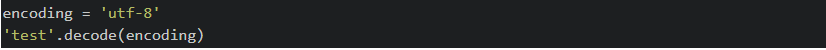# Python - Convert bytes to stringbytes, python, python bytes to string, string

Converting bytes to a string in Python can be done by decoding the bytes into a unicode string. Below are a few examples of how to convert bytes to a string in both Python 2 and Python 3. We hope this quick guide helps the next time you are converting byte values in Python! If you need to convert int to string, check out our other Python blog post.

### Python 2 - Convert bytes to string

In Python 2 you are able to convert the bytes to a string using either the decode() or unicode() methods. Check out the example below.

``````encoding = 'utf-8'
'test'.decode(encoding)``````
``unicode('test', encoding)``

### Python 3 - Convert bytes to string

Python 3 also has two options to convert the bytes to a string, although instead of using the unicode() method we now use the str() method. See examples below:

``````encoding = 'utf-8'
b'test'.decode(encoding)``````
``str(b'test', encoding)``

shop